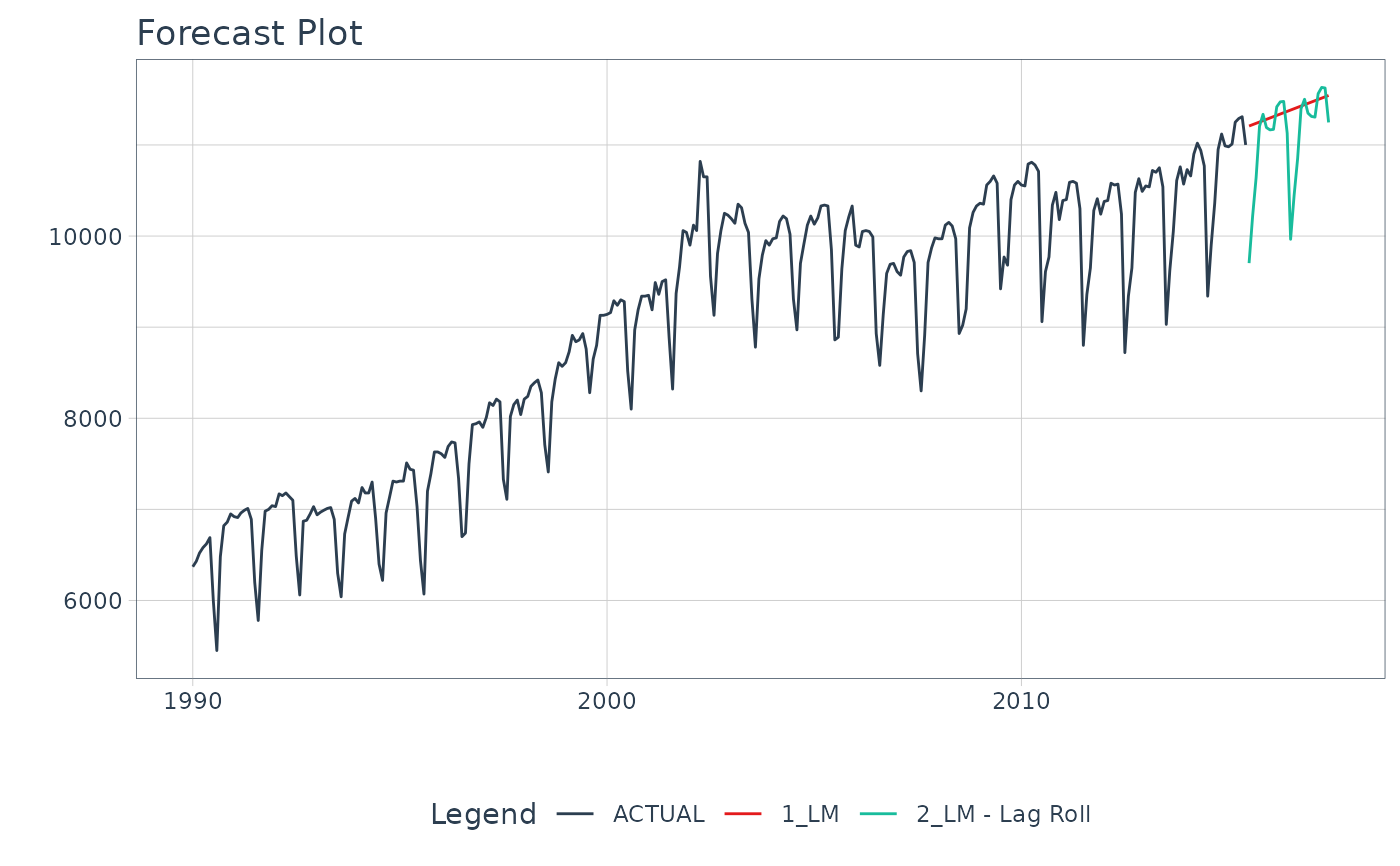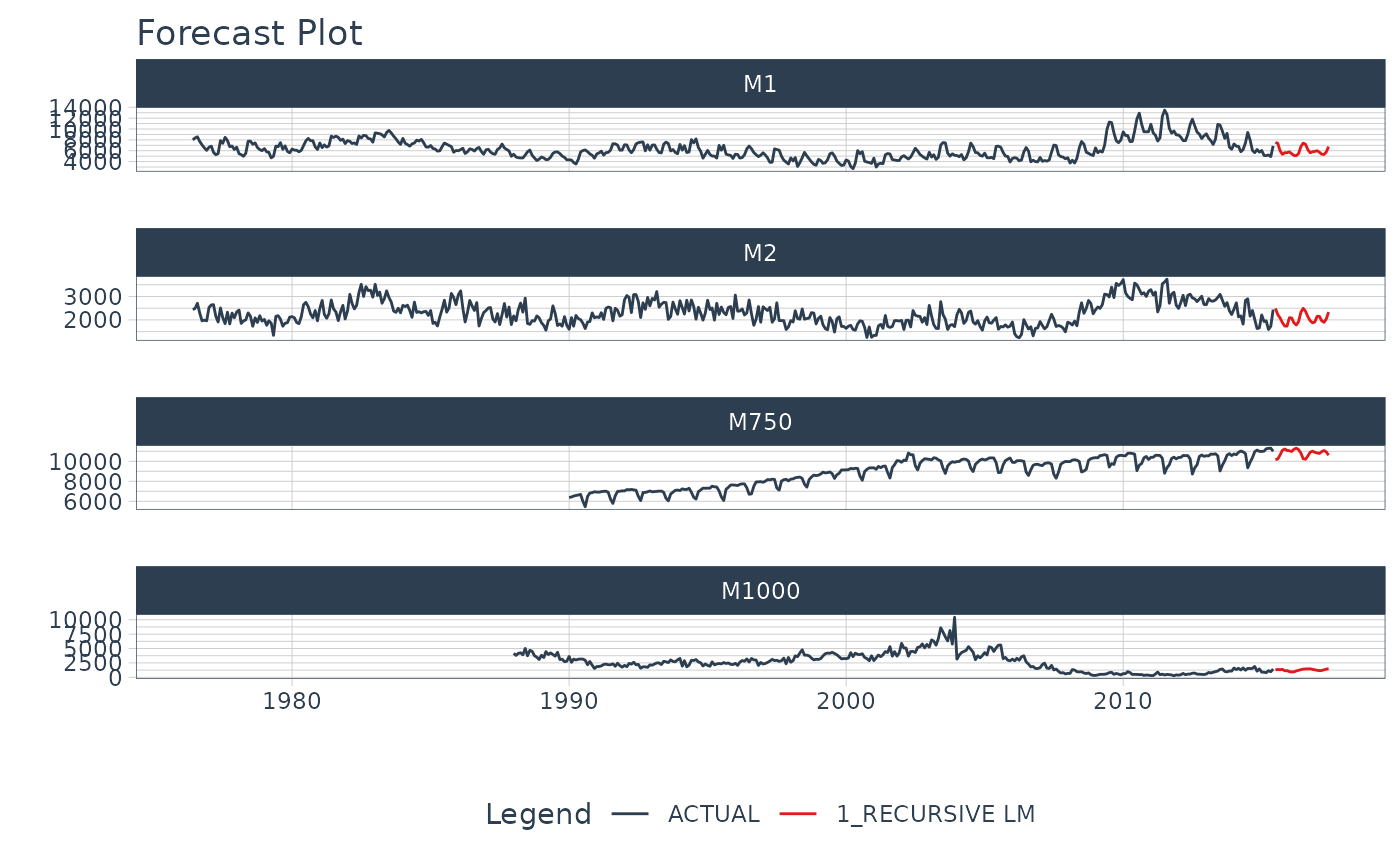Create a Recursive Time Series Model from a Parsnip or Workflow Regression Model

## Usage

recursive(object, transform, train_tail, id = NULL, chunk_size = 1, ...)

## Arguments

object

An object of model_fit or a fitted workflow class

transform

A transformation performed on new_data after each step of recursive algorithm.

• Transformation Function: Must have one argument data (see examples)

train_tail

A tibble with tail of training data set. In most cases it'll be required to create some variables based on dependent variable.

id

(Optional) An identifier that can be provided to perform a panel forecast. A single quoted column name (e.g. id = "id").

chunk_size

The size of the smallest lag used in transform. If the smallest lag necessary is n, the forecasts can be computed in chunks of n, which can dramatically improve performance. Defaults to 1. Non-integers are coerced to integer, e.g. chunk_size = 3.5 will be coerced to integer via as.integer().

...

Not currently used.

## Value

An object with added recursive class

## Details

What is a Recursive Model?

A recursive model uses predictions to generate new values for independent features. These features are typically lags used in autoregressive models. It's important to understand that a recursive model is only needed when the Lag Size < Forecast Horizon.

Why is Recursive needed for Autoregressive Models with Lag Size < Forecast Horizon?

When the lag length is less than the forecast horizon, a problem exists were missing values (NA) are generated in the future data. A solution that recursive() implements is to iteratively fill these missing values in with values generated from predictions.

Recursive Process

When producing forecast, the following steps are performed:

1. Computing forecast for first row of new data. The first row cannot contain NA in any required column.

2. Filling i-th place of the dependent variable column with already computed forecast.

3. Computing missing features for next step, based on already calculated prediction. These features are computed with on a tibble object made from binded train_tail (i.e. tail of training data set) and new_data (which is an argument of predict function).

4. Jumping into point 2., and repeating rest of steps till the for-loop is ended.

Recursion for Panel Data

Panel data is time series data with multiple groups identified by an ID column. The recursive() function can be used for Panel Data with the following modifications:

1. Supply an id column as a quoted column name

2. Replace tail() with panel_tail() to use tails for each time series group.

• panel_tail() - Used to generate tails for multiple time series groups.

## Examples


# \donttest{
# Libraries & Setup ----
library(modeltime)
library(tidymodels)
library(tidyverse)
library(lubridate)
library(timetk)
library(slider)

# ---- SINGLE TIME SERIES (NON-PANEL) -----

m750
#> # A tibble: 306 × 3
#>    id    date       value
#>    <fct> <date>     <dbl>
#>  1 M750  1990-01-01  6370
#>  2 M750  1990-02-01  6430
#>  3 M750  1990-03-01  6520
#>  4 M750  1990-04-01  6580
#>  5 M750  1990-05-01  6620
#>  6 M750  1990-06-01  6690
#>  7 M750  1990-07-01  6000
#>  8 M750  1990-08-01  5450
#>  9 M750  1990-09-01  6480
#> 10 M750  1990-10-01  6820
#> # … with 296 more rows

FORECAST_HORIZON <- 24

m750_extended <- m750 %>%
group_by(id) %>%
future_frame(
.length_out = FORECAST_HORIZON,
.bind_data  = TRUE
) %>%
ungroup()
#> .date_var is missing. Using: date

# TRANSFORM FUNCTION ----
# - Function runs recursively that updates the forecasted dataset
lag_roll_transformer <- function(data){
data %>%
# Lags
tk_augment_lags(value, .lags = 1:12) %>%
# Rolling Features
mutate(rolling_mean_12 = lag(slide_dbl(
value, .f = mean, .before = 12, .complete = FALSE
), 1))
}

# Data Preparation
m750_rolling <- m750_extended %>%
lag_roll_transformer() %>%
select(-id)

train_data <- m750_rolling %>%
drop_na()

future_data <- m750_rolling %>%
filter(is.na(value))

# Modeling

# Straight-Line Forecast
model_fit_lm <- linear_reg() %>%
set_engine("lm") %>%
# Use only date feature as regressor
fit(value ~ date, data = train_data)

# Autoregressive Forecast
model_fit_lm_recursive <- linear_reg() %>%
set_engine("lm") %>%
# Use date plus all lagged features
fit(value ~ ., data = train_data) %>%
# Add recursive() w/ transformer and train_tail
recursive(
transform  = lag_roll_transformer,
train_tail = tail(train_data, FORECAST_HORIZON)
)

model_fit_lm_recursive
#> Recursive [parsnip model]
#>
#> parsnip model object
#>
#>
#> Call:
#> stats::lm(formula = value ~ ., data = data)
#>
#> Coefficients:
#>     (Intercept)             date       value_lag1       value_lag2
#>       147.32008          0.01273          1.59298          0.76666
#>      value_lag3       value_lag4       value_lag5       value_lag6
#>         0.73081          0.76950          0.76871          0.74755
#>      value_lag7       value_lag8       value_lag9      value_lag10
#>         0.77872          0.72985          0.75257          0.76582
#>     value_lag11      value_lag12  rolling_mean_12
#>         0.79979          1.62469         -9.85822
#>

# Forecasting
modeltime_table(
model_fit_lm,
model_fit_lm_recursive
) %>%
update_model_description(2, "LM - Lag Roll") %>%
modeltime_forecast(
new_data    = future_data,
actual_data = m750
) %>%
plot_modeltime_forecast(
.interactive        = FALSE,
.conf_interval_show = FALSE
)# MULTIPLE TIME SERIES (PANEL DATA) -----

m4_monthly
#> # A tibble: 1,574 × 3
#>    id    date       value
#>    <fct> <date>     <dbl>
#>  1 M1    1976-06-01  8000
#>  2 M1    1976-07-01  8350
#>  3 M1    1976-08-01  8570
#>  4 M1    1976-09-01  7700
#>  5 M1    1976-10-01  7080
#>  6 M1    1976-11-01  6520
#>  7 M1    1976-12-01  6070
#>  8 M1    1977-01-01  6650
#>  9 M1    1977-02-01  6830
#> 10 M1    1977-03-01  5710
#> # … with 1,564 more rows

FORECAST_HORIZON <- 24

m4_extended <- m4_monthly %>%
group_by(id) %>%
future_frame(
.length_out = FORECAST_HORIZON,
.bind_data  = TRUE
) %>%
ungroup()
#> .date_var is missing. Using: date

# TRANSFORM FUNCTION ----
# - NOTE - We create lags by group
lag_transformer_grouped <- function(data){
data %>%
group_by(id) %>%
tk_augment_lags(value, .lags = 1:FORECAST_HORIZON) %>%
ungroup()
}

m4_lags <- m4_extended %>%
lag_transformer_grouped()

train_data <- m4_lags %>%
drop_na()

future_data <- m4_lags %>%
filter(is.na(value))

# Modeling Autoregressive Panel Data
model_fit_lm_recursive <- linear_reg() %>%
set_engine("lm") %>%
fit(value ~ ., data = train_data) %>%
recursive(
id         = "id", # We add an id = "id" to specify the groups
transform  = lag_transformer_grouped,
# We use panel_tail() to grab tail by groups
train_tail = panel_tail(train_data, id, FORECAST_HORIZON)
)

modeltime_table(
model_fit_lm_recursive
) %>%
modeltime_forecast(
new_data    = future_data,
actual_data = m4_monthly,
keep_data   = TRUE
) %>%
group_by(id) %>%
plot_modeltime_forecast(
.interactive = FALSE,
.conf_interval_show = FALSE
)# }